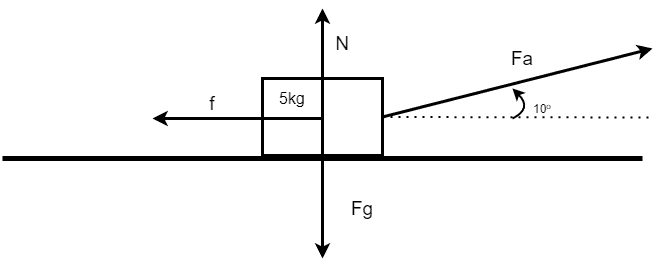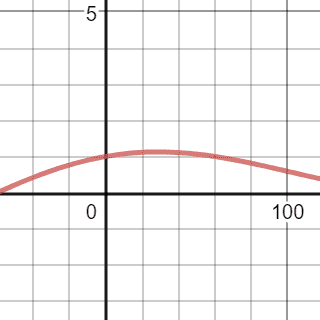# Change in acceleration with change of angle of applied force

GreenOlive
Homework Statement:
How will the acceleration of the block change if the angle of the applied force is increase by 5 degrees? Write Increase, Decrease or Stay the same.
Relevant Equations:
Newton 2, Fnet = ma
friction = N * μLet ##μ_k## = 0.5
##F_a## = 10 Newtons
##\theta## is the angel of the Applied force.
How will the acceleration of the block change if the angle of the applied force is increase by ##5^o##? Write Increase, Decrease or Stay the same.

Recently we were discussing a question similar to this in class, I believe the acceleration should INCREASE however the memorandum for the question said it would DECREASE. I would like to just confirm my maths is correct (This is not the first time I have seen such a question).

This is my general solution.

Let right be positive.

$$F_{net} = ma$$
$$F_{net} = F_{a,x} - f$$
$$ma = F_{a,x} - (N \times μ_k)$$
$$ma = F_a \times cos\theta - ((F_g-F_{a,y}) \times μ_k)$$
$$ma = F_a \times cos\theta - ((F_g-(F_a\times sin\theta)) \times μ_k)$$

As ##\theta## is the only variable changing let's isolate the expressions it is part of.

$$ma = F_a \times cos\theta + F_a\times sin\theta \times μ_k - F_g \times μ_k$$
$$ma = F_a (cos\theta + sin\theta \times μ_k) - F_g \times μ_k$$

lets graph ##(cos\theta + sin\theta \times μ_k) ##Desmos graph

Looking at the graph we can see ##(cos\theta + sin\theta \times μ_k)##increases from 0 until its maximum at 28.955 degrees from which it starts to decrease.
And as $$a = \frac{F_a (cos\theta + sin\theta \times μ_k) - F_g \times μ_k}m$$

Therefore by increasing the angle of the applied force by 5 degrees the acceleration will INCREASE.
By looking closer at the graph you could see that ##\theta## would need to be greater than 48.76 degrees for the acceleration to Descrease(or less than 5).

Who is Incorrect, the Memo or I?

Last edited by a moderator:

Gold Member
Your graph, if proportional to acceleration, still shows positive acceleration when θ is > 90 degrees, which seems to imply that the block will move forward when you're applying negative force to it. That intuitively suggests something amiss.
It being positive for a while with a negative θ is OK. A force pulling a bit down can still move the block until the added friction eventually prevents any motion. So the left side of the graph seems ok.

GreenOlive
Can we create a limit: -90 < ##\theta## < 90. Angels not in this range would imply a negative applied force and then positive friction(if right is still positive) so you would have to change the equation.

Homework Helper
Gold Member
••